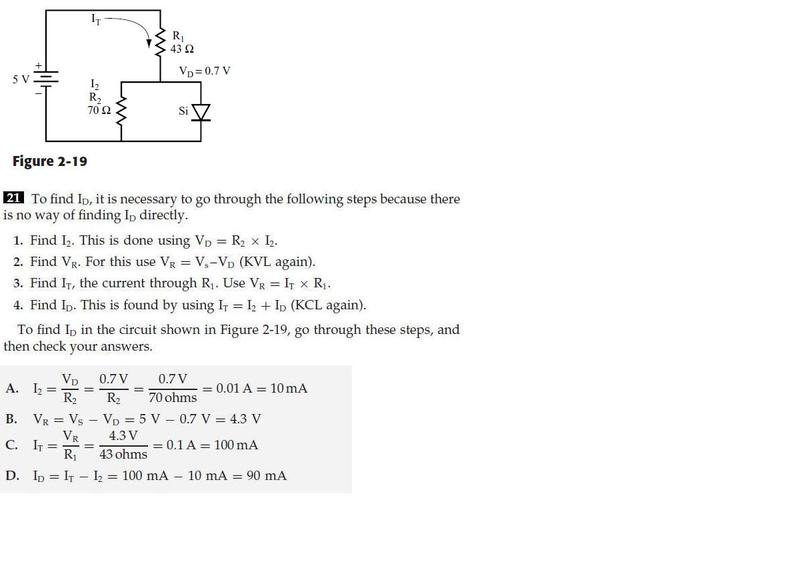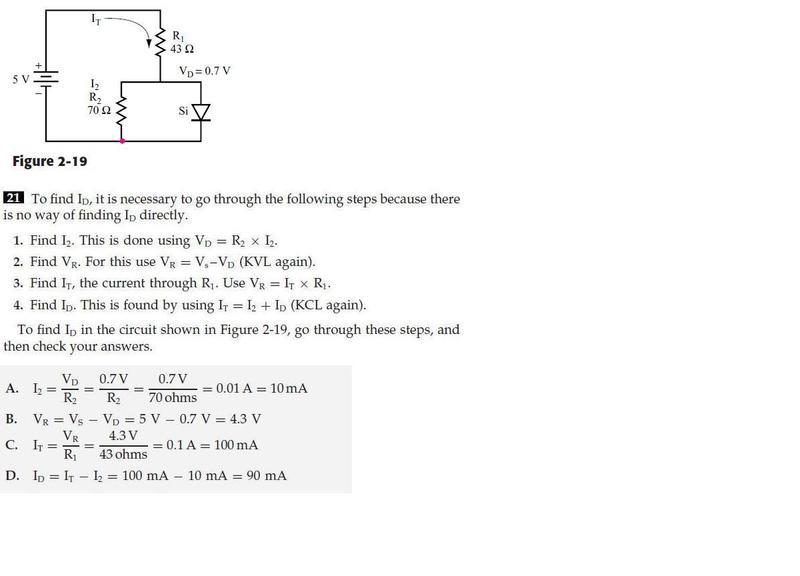# Help needed with a diode and resistor formula

• Eisen

#### Eisen

This isn't a homework question but it is a formula from a book I have been using to study, so I'm posting it here.

Here is the circuit and formula -I don't understand why the voltage drop of 0.7 volts across the diode is being used as part of the formula to work out the current across R2 when R2 has it's own voltage drop across it. Can someone explain this to me please?

I think that since the diode and resistor are in parallel, the voltage drop across them has to be the same. So since you know the voltage drop across the diode you can just extend that to R2.

I don't see why the voltage drop across R2 and the diode would be the same.

I don't see why the voltage drop across R2 and the diode would be the same.

Well, the diode and the resistor are connected. The voltage at the pink point I added to your diagram can only be one value.So the voltage drop through resistor 2 is the same as in the diode because they are in parallel?

So the voltage drop through resistor 2 is the same as in the diode because they are in parallel?

Yes. ANY components that are in parallel all share the same potential drop.

Thanks, well that clears things up for me.